## Annotation

If you take any sum, then all other sums can be divided into three groups: identical sums, decomposition sums, and other sums. The classification criteria are the terms and the result of the addition.

## Identical sums

Identical sums are combined into a separate group by the commutativity property. Sums from this group have pairwise identical terms and an equal number of terms. The result of adding these sums is the same. Here is an example of identical sums.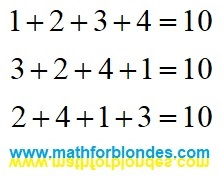Identical sums

The number of sums in this group is determined by the number of terms. For infinite sums, it is equal to infinity.

## Decomposition sums

If the results of adding a different number of terms or terms of different sizes are the same, then such sums form a decomposition group.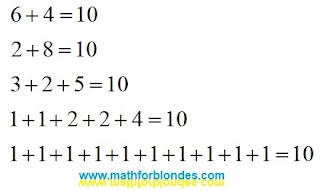Decomposition sums

Any of these sums can be obtained by decomposing the result of addition into terms using linear angular functions. Having thus obtained two terms, any of them can also be decomposed into terms, and so on. An example expansion for the first three sums: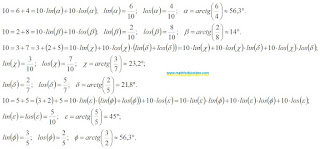Decomposing into terms

An example of decomposing a number into three terms shows that different decomposition options can give the same sum, which underlies the associative properties of addition. The more terms the sum contains, the more different expansion options there can be. The expansion into terms can be continued indefinitely. Different angles and different decomposition algorithms make it possible to obtain different variants of infinite sums. The theory of limits allows you to determine the result of addition based on the analysis of terms. The expansion into terms allows the summation result to be represented as an infinite series of terms.

For example, let's decompose the unit into an infinite sum according to the following principle: the expansion is performed at an angle of 45°, each second term is decomposed into two terms.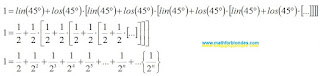Decomposition into an infinite number of terms

The curly brackets indicate the sum of the invisible compensating group of terms, which complements the result of addition to an integer unit. It can be assumed that any sum, even an infinite divergent series, in trigonometric form is equal to one.

## Other sums

If the result of adding any amount differs from the result of adding the amount in question, then this amount has nothing to do with the amount in question and belongs to the “other sums” group.

Let's assume that for the sum a+b=c there is another addition result d that is not equal to c. That is, a+b=d. We represent these two expressions using linear angle functions, and then we translate them into a trigonometric form: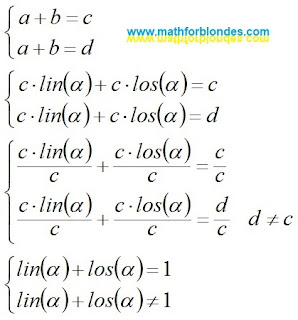Different addition results

The assumption that there are different addition results for the same sum takes us beyond the boundaries of mathematics, where the basic trigonometric relations stop working: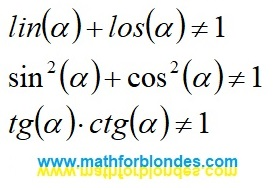Beyond the boundaries of mathematics

Why can't the same sum of terms have two different addition results? You can understand this by considering the reverse process - the transformation of trigonometric functions into specific mathematical sums. I will cover this in more detail in a separate post.

In conclusion of the conversation about the presence of two different results of addition in divergent series, I will give an example from physics. In the earth's crust (convergent series) there are natural caves (the sum of the convergent series). Using special mechanisms (convergence according to Cesaro, etc.) we can get artificial tunnels (the sum of a convergent series). There are no natural caves in the seas and oceans (divergent series, the sum of the series is missing). The use of special mechanisms (convergence according to Cesaro, etc.) allows us to obtain artificial tunnels (the sum of a divergent series) in the water column. Based on this mathematically proven theory, it is possible to design a network of tunnels for roads and railways that encircle the entire earth's surface. Such a theory is quite possible if we do not understand the difference between solids (convergent series) and liquids (divergent series).

## Conclusion

There are no mathematical methods that allow you to get a different addition result for the sum in question.